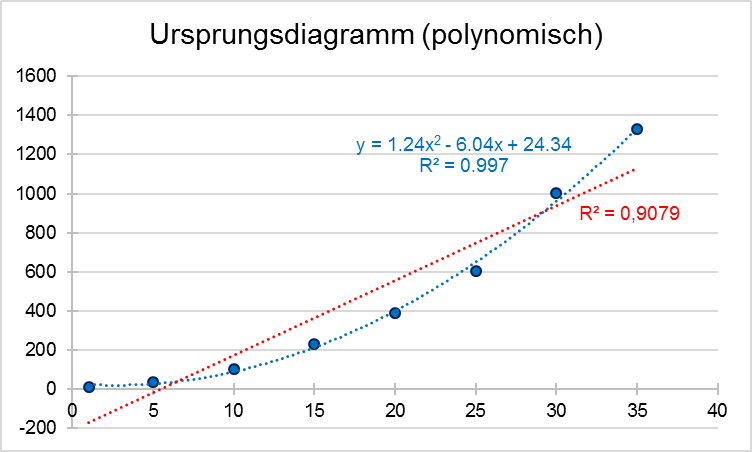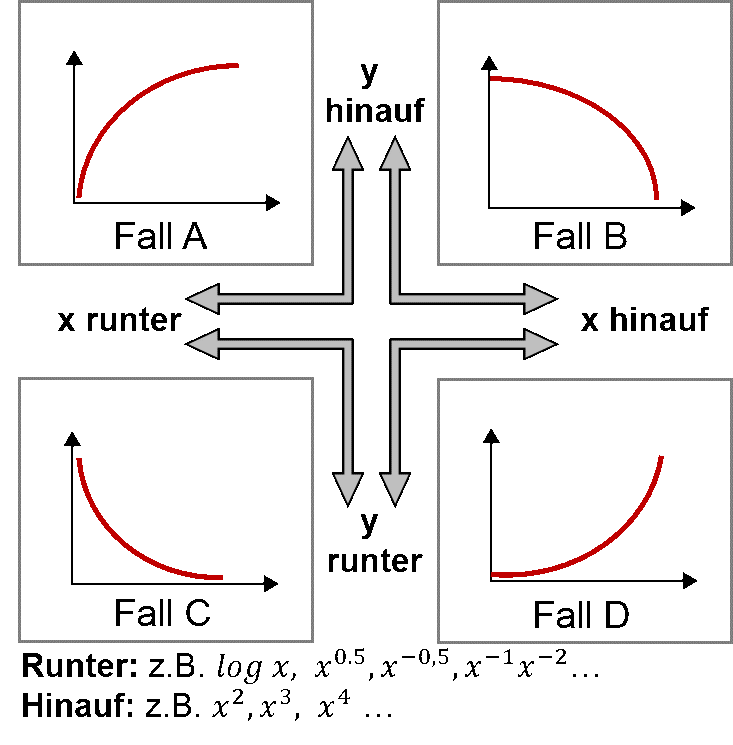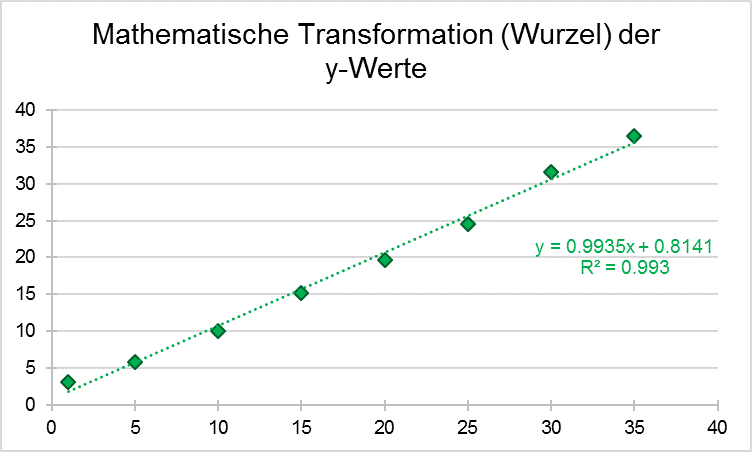# Is the polynomial regression linear or non-linear

## Linear versus non-linear regression: what should be considered?

In every method that involves the quantification of an analyte of a drug or its active ingredient, the linearity of a calibration line is a decisive criterion for the correctness of the values. In the best case, it should be possible to display the measured values ​​in direct proportion to the concentration used. Most measurement methods have their limits, which is why the measurable range (linear range) is often limited. According to the ICH Q2 (R1) guide for method validation, the linearity is in a specified working range (Range) using the least squares method. It is essential to avoid imposing a linear regression on the data generated, where it may not be available. If a curve can be guessed at when plotting the concentration against the measured values, a more precise regression analysis should be considered.

### Linear or non-linear: Correlation coefficients provide information

If a very shallow curve can be seen, the decision as to whether linear regression can be safely applied is in the eye of the beholder. The calculation of two correlation coefficients (R) can help here. The Pearson correlation coefficient only considers linear relationships. For example, if it is significantly less than 0.95, it may either be too wide a spread of the measurement results or a non-linear correlation. Certainty can be obtained through the so-called Spearman correlation coefficient. This takes into account both linear and non-linear correlations. If both coefficients are calculated and a significantly higher value is obtained for the Spearman coefficient than for the Pearson coefficient, then in all probability it is a non-linear correlation. If both correlation coefficients are almost identical, it is a linear correlation.

### Transformation of the raw data

In the case of a non-linear dependency, the ICH Q2 (R1) guide suggests a mathematical transformation of the raw data in order to create a suitable linear regression. But what is the best way to do it?

In our example we have generated fictitious values ​​that follow a polynomial function of the second degree (y = ax2 + bx + c). The graphic representation can be seen in the first figure (blue values).Here it becomes clear that linear regression cannot be used with a clear conscience. If you try it anyway, you get a coefficient of determination (R2) of 0.908. A polynomial function, on the other hand, can be inserted very well (blue line). A simple and easy to use method to bring the polynomial function into a linear dependency describes the so-called "power ladder". Depending on the type of curve curvature, the power ladder can be used to determine the changes that must be made to the x or y variables in order to approach a linear dependency (see the following figure):If, as in our example, there is a rising curve (ie “case D” in the figure above), the x variable can be raised to the power by squaring the values ​​or taking them to the power of 3. Another approach would be to raise the y-variable to the power, for example, using a logarithm function or a square root. So one can gradually approach a linearity. In our example we decided to apply the root function to the y variables (= y0,5) and come to the following result:After the corresponding mathematical transformations have been carried out, linear regression can be used with the newly acquired x and y values ​​and the coefficient of determination (R2) be calculated. In our example we arrive at a good linear relationship with a coefficient of determination of 0.993. New measured values ​​that are to be analyzed using the transformed linear regression must be subjected to the same mathematical transformation before they are analyzed. In our case, the roots of all newly measured y-values ​​must be taken. Then they can be used as usual using the regression equation and solved for x.

Tags: method validationICH Q2 (R1) non-linear regressionLinear regressionLinearity